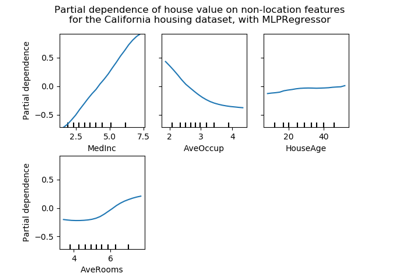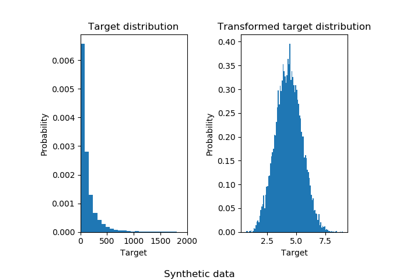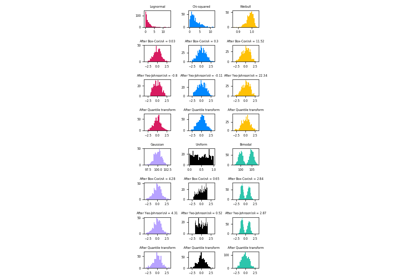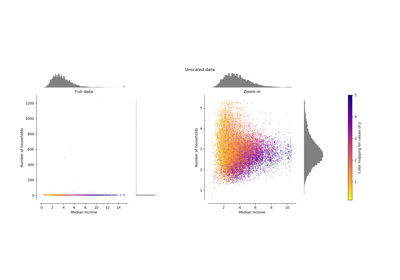# sklearn.preprocessing.QuantileTransformer¶

class sklearn.preprocessing.QuantileTransformer(n_quantiles=1000, output_distribution='uniform', ignore_implicit_zeros=False, subsample=100000, random_state=None, copy=True)[source]

Transform features using quantiles information.

This method transforms the features to follow a uniform or a normal distribution. Therefore, for a given feature, this transformation tends to spread out the most frequent values. It also reduces the impact of (marginal) outliers: this is therefore a robust preprocessing scheme.

The transformation is applied on each feature independently. First an estimate of the cumulative distribution function of a feature is used to map the original values to a uniform distribution. The obtained values are then mapped to the desired output distribution using the associated quantile function. Features values of new/unseen data that fall below or above the fitted range will be mapped to the bounds of the output distribution. Note that this transform is non-linear. It may distort linear correlations between variables measured at the same scale but renders variables measured at different scales more directly comparable.

Read more in the User Guide.

New in version 0.19.

Parameters
n_quantilesint, optional (default=1000 or n_samples)

Number of quantiles to be computed. It corresponds to the number of landmarks used to discretize the cumulative distribution function. If n_quantiles is larger than the number of samples, n_quantiles is set to the number of samples as a larger number of quantiles does not give a better approximation of the cumulative distribution function estimator.

output_distributionstr, optional (default=’uniform’)

Marginal distribution for the transformed data. The choices are ‘uniform’ (default) or ‘normal’.

ignore_implicit_zerosbool, optional (default=False)

Only applies to sparse matrices. If True, the sparse entries of the matrix are discarded to compute the quantile statistics. If False, these entries are treated as zeros.

subsampleint, optional (default=1e5)

Maximum number of samples used to estimate the quantiles for computational efficiency. Note that the subsampling procedure may differ for value-identical sparse and dense matrices.

random_stateint, RandomState instance or None, optional (default=None)

If int, random_state is the seed used by the random number generator; If RandomState instance, random_state is the random number generator; If None, the random number generator is the RandomState instance used by np.random. Note that this is used by subsampling and smoothing noise.

copyboolean, optional, (default=True)

Set to False to perform inplace transformation and avoid a copy (if the input is already a numpy array).

Attributes
n_quantiles_integer

The actual number of quantiles used to discretize the cumulative distribution function.

quantiles_ndarray, shape (n_quantiles, n_features)

The values corresponding the quantiles of reference.

references_ndarray, shape(n_quantiles, )

Quantiles of references.

quantile_transform

Equivalent function without the estimator API.

PowerTransformer

Perform mapping to a normal distribution using a power transform.

StandardScaler

Perform standardization that is faster, but less robust to outliers.

RobustScaler

Perform robust standardization that removes the influence of outliers but does not put outliers and inliers on the same scale.

Notes

NaNs are treated as missing values: disregarded in fit, and maintained in transform.

For a comparison of the different scalers, transformers, and normalizers, see examples/preprocessing/plot_all_scaling.py.

Examples

>>> import numpy as np
>>> from sklearn.preprocessing import QuantileTransformer
>>> rng = np.random.RandomState(0)
>>> X = np.sort(rng.normal(loc=0.5, scale=0.25, size=(25, 1)), axis=0)
>>> qt = QuantileTransformer(n_quantiles=10, random_state=0)
>>> qt.fit_transform(X)
array([...])


Methods

 fit(self, X[, y]) Compute the quantiles used for transforming. fit_transform(self, X[, y]) Fit to data, then transform it. get_params(self[, deep]) Get parameters for this estimator. inverse_transform(self, X) Back-projection to the original space. set_params(self, \*\*params) Set the parameters of this estimator. transform(self, X) Feature-wise transformation of the data.
__init__(self, n_quantiles=1000, output_distribution='uniform', ignore_implicit_zeros=False, subsample=100000, random_state=None, copy=True)[source]

Initialize self. See help(type(self)) for accurate signature.

fit(self, X, y=None)[source]

Compute the quantiles used for transforming.

Parameters
Xndarray or sparse matrix, shape (n_samples, n_features)

The data used to scale along the features axis. If a sparse matrix is provided, it will be converted into a sparse csc_matrix. Additionally, the sparse matrix needs to be nonnegative if ignore_implicit_zeros is False.

Returns
selfobject
fit_transform(self, X, y=None, **fit_params)[source]

Fit to data, then transform it.

Fits transformer to X and y with optional parameters fit_params and returns a transformed version of X.

Parameters
Xnumpy array of shape [n_samples, n_features]

Training set.

ynumpy array of shape [n_samples]

Target values.

**fit_paramsdict

Returns
X_newnumpy array of shape [n_samples, n_features_new]

Transformed array.

get_params(self, deep=True)[source]

Get parameters for this estimator.

Parameters
deepbool, default=True

If True, will return the parameters for this estimator and contained subobjects that are estimators.

Returns
paramsmapping of string to any

Parameter names mapped to their values.

inverse_transform(self, X)[source]

Back-projection to the original space.

Parameters
Xndarray or sparse matrix, shape (n_samples, n_features)

The data used to scale along the features axis. If a sparse matrix is provided, it will be converted into a sparse csc_matrix. Additionally, the sparse matrix needs to be nonnegative if ignore_implicit_zeros is False.

Returns
Xtndarray or sparse matrix, shape (n_samples, n_features)

The projected data.

set_params(self, **params)[source]

Set the parameters of this estimator.

The method works on simple estimators as well as on nested objects (such as pipelines). The latter have parameters of the form <component>__<parameter> so that it’s possible to update each component of a nested object.

Parameters
**paramsdict

Estimator parameters.

Returns
selfobject

Estimator instance.

transform(self, X)[source]

Feature-wise transformation of the data.

Parameters
Xndarray or sparse matrix, shape (n_samples, n_features)

The data used to scale along the features axis. If a sparse matrix is provided, it will be converted into a sparse csc_matrix. Additionally, the sparse matrix needs to be nonnegative if ignore_implicit_zeros is False.

Returns
Xtndarray or sparse matrix, shape (n_samples, n_features)

The projected data.

## Examples using sklearn.preprocessing.QuantileTransformer¶